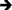## ▼ == Equals

Tag Link Category == Equals Symbol Symbol No Preferred 5.0 Unchanged Any Boolean None LCAPI Lasso 8.5, Lasso 8.0, Lasso 7.0, Lasso 6.0, Lasso 5.0

## ▼ Description

== checks to see if the value of the right parameter is equal to the value of the left parameter. It returns True if they are or False otherwise.

Note: If the parameter on the right hand side of the symbol is a negative literal it should be surrounded by parentheses. For example, (4 == (-1)).

Note that strings are compared without taking case into account. For a case sensitive comparison use [String->Equals] with the optional -Case parameter.

## ▼ Syntax

[If: Left_Parameter == Right_Parameter]
...
[/If]

<?LassoScript
If: Left_Parameter == Right_Parameter;
...
/If;
?>

## ▼ Parameters

Required Parameters
Left_Parameter The value to be compared.
Right_Parameter The value to be compared.

## ▼ Examples

To return a result if one value equals another:

Use the equals symbol == within an opening [If] tag. The following example checks if the string '100' equals the integer 100. The contents of the [If] ... [/If] tags is returned since the condition is True.

[If: '100' == 100]
The string and integer are equal.
[/If]The string and integer are equal.